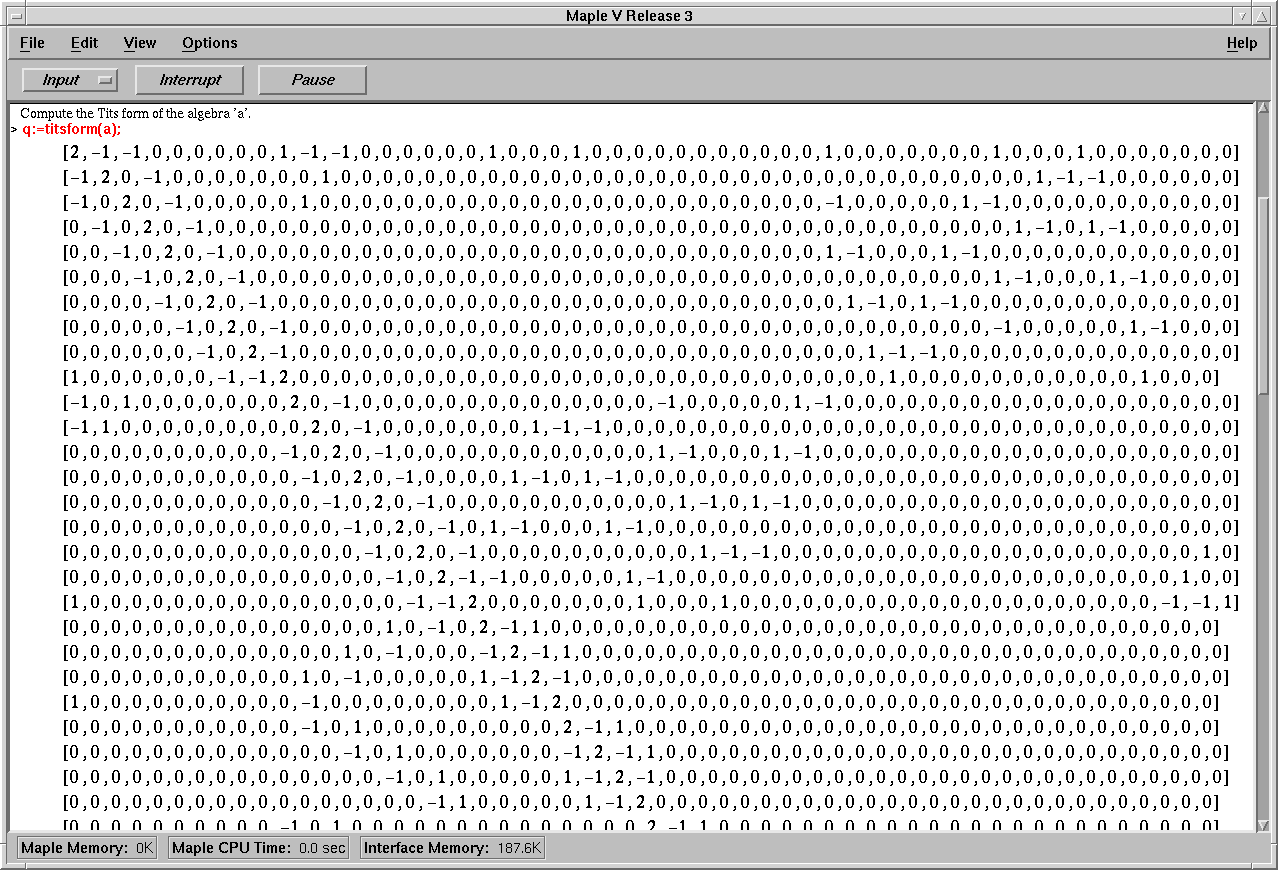### The Tits form

The Tits form qa is an integral quadratic form associated with an algebra a. In particular, qa is a linear map from Zn to the integers Z where n is the number of vertices in the quiver of the algebra a. Such a quadratic form is represented in CREP by its integral symmetric coefficient matrix, ie a matrix Q with integral coefficients and the property qa(x) = xT Q x for any vector x in Zn.

The function 'titsform' calculates the corresponding matrix for a given bound poset algebra. This matrix in our example being of size 54 x 54 we display only part of the function's output:Back to the worksheet of the example session.